Courses

# Test: Field Effect Transistors

## 10 Questions MCQ Test Topicwise Question Bank for Electrical Engineering | Test: Field Effect Transistors

Description
This mock test of Test: Field Effect Transistors for Electrical Engineering (EE) helps you for every Electrical Engineering (EE) entrance exam. This contains 10 Multiple Choice Questions for Electrical Engineering (EE) Test: Field Effect Transistors (mcq) to study with solutions a complete question bank. The solved questions answers in this Test: Field Effect Transistors quiz give you a good mix of easy questions and tough questions. Electrical Engineering (EE) students definitely take this Test: Field Effect Transistors exercise for a better result in the exam. You can find other Test: Field Effect Transistors extra questions, long questions & short questions for Electrical Engineering (EE) on EduRev as well by searching above.
QUESTION: 1

### FET is advantageous in comparison with BJT because of

Solution:

As the input circuit of FET is reversed biased, FET exhibits a much higher input impedance (in the order of 100 MΩ) and lower output impedance. So, FET can act as an excellent buffer amplifier but the BJT has low input impedance because its input circuit is forward biased.

QUESTION: 2

Solution:
QUESTION: 3

### JFET has main drawback of

Solution:

JFET has main drawback of having small gain bandwidth product due to the junction capacitive effects and produce more signal distortion except for small signal operation.

QUESTION: 4

The extremely high input impedance of a MOSFET is primarily due to the

Solution:
QUESTION: 5

In a JFET, at pinch-off voltage applied on the gate

Solution:

The “drain characteristic” or the “output characteristic” is shown below. Here, in the pinch-off region, as VDS is increased then ID almost remains constant.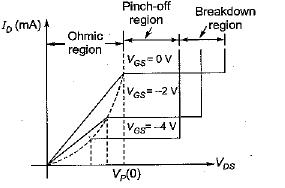QUESTION: 6

What is the value of transconductance when the reverse gate voltage of JFET changes from 4.0 to 3.9 V and the drain current changes from 1.3 to 1.6 mA?

Solution: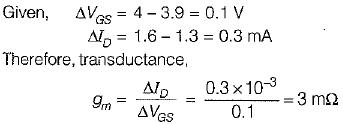QUESTION: 7

A FET has a drain current of 4 mA. If IDss = 8 mA and VGS(off) = - 6V, then the value of gate to source voltage would be

Solution: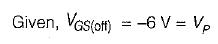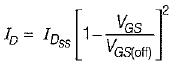or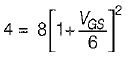or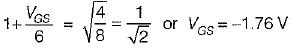QUESTION: 8

The gate controls

Solution:
QUESTION: 9

Which of the following statements associated with FET is/are correct compared to BJT?
1. It has higher cut-off frequencies and switching speed.
2. The performance of FET is relatively more affected by ambient temperature changes.
3. FETs are cheaper to produce.
4. FETs are suitable for the fabrication of ICs because they occupy less space than BJTs.

Solution:
• Since FET does not suffer from minority carrier storage effects, it has higher switching speeds and cut-off frequencies.'
• The performance of FET is relatively unaffected by ambient temperature changes compared to BJT.
• BJTs are cheaper to produce.
• FETs are much easier to fabricate and are particularly suitable for ICs because they occupy less space than BJTs.

Hence, statements 1 and 4 are only correct.

QUESTION: 10

Assertion (A): FET does not suffer from thermal breakdown.
Reason (R): FET has a positive temperature coefficient for resistance.

Solution: